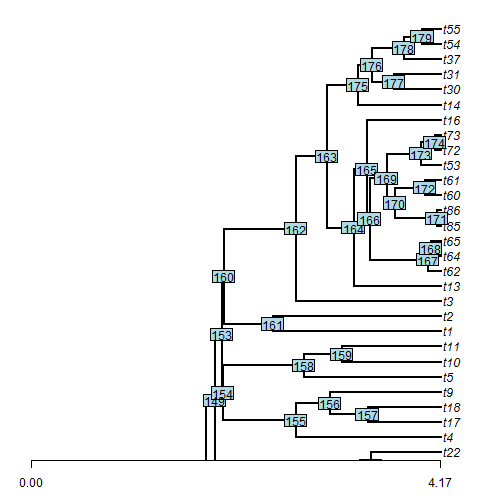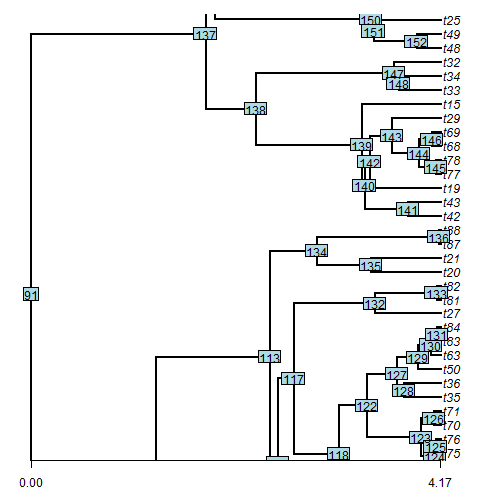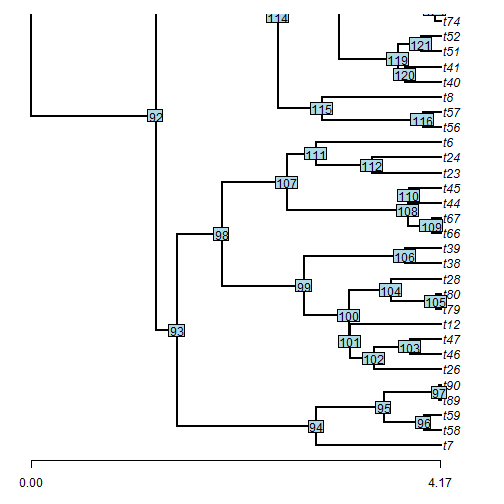## Thursday, March 5, 2015

### Splitting a tree across multiple pages, with node labels & other such things

After posting a simple wrapper to split a plotted tree across plotting devices or pages of a PDF file, I received the following inquiry:

Would there be a way to modify this so that annotations like node labels and axes could be applied to both 1/2s of a split tree at the same time? I've been struggling with a similar problem using plot.phylo() and we can get our tree to look like what we want on one page, but splitting it up while keeping node and branch annotations etc is problematic.

This seems hard - but is actually not too difficult. I can just add an argument `fn` that is a function containing the functions we want to run for each page of our plotted tree.

First, here is the function:

``````split.plotTree<-function(tree,splits=NULL,file=NULL,fn,...){
ef<-0.037037037037
if(!is.null(file)) pdf(file,width=8.5,height=11)
if(is.null(splits)) splits<-(floor(0.5*Ntip(tree))+0.5)/Ntip(tree)
S<-matrix(c(0,splits,splits,1+1/Ntip(tree)),length(splits)+1,2)
S<-cbind(S[,1]+ef*(S[,2]-S[,1]),S[,2]-ef*(S[,2]-S[,1]))
for(i in nrow(S):1){
plotTree(tree,ylim=Ntip(tree)*S[i,],...)
fn()
}
if(!is.null(file)) oo<-dev.off()
}
``````

Now, just for example, let's create a custom function that adds an axis to each subplot, and includes node labels:

``````foo<-function(){
nodelabels()
axis(1,at=c(0,round(max(nodeHeights(tree)),2)))
}
``````

Finally, let's load phytools, simulate a tree, and try it out:

``````library(phytools)
tree<-pbtree(n=90)
split.plotTree(tree,splits=c(0.3275,0.683),fn=foo,ftype="i",
mar=c(3,1,1,1))
``````That's all there is to it.